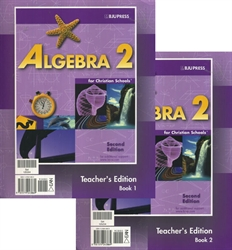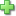# Algebra 2 - Teacher Edition (old)

Spiralbound, 866 pages
Current Retail Price: \$78.61
Not in stock

Review basic algebraic functions and extend your student's skills in graphing and in solving equations. Introduce exponential functions, logarithms, and trigonometry. Present more advanced topics such as complex numbers, rational expressions and equations, conic sections, and probability and statistics. A matrix algebra feature, Algebra Around the World, Algebra and Scripture, dominion modeling activities for graphing calculators, and biographical sketches of mathematicians are included.

The colorful Teacher Edition includes student pages with helpful presentation notes and answers (including solutions) as well as sections with motivational ideas, common student errors, and more.

Did you find this review helpful?
Series DescriptionClick here to write a review